# #701. 关羽下象棋

1. 卒初始在第 $x_1$ 行第 $y_1$ 列的格点上，车初始在第 $x_2$ 行第 $y_2$ 列的格点上。
2. 卒每次可以在左、右、下三种移动方向中选择一种，然后移动一格（但是不能往上）。即，若记第 $x$ 行第 $y$ 列的格点为 $(x,y)$，则卒可以从 $(x,y)$ 移动到 $(x+1,y),(x,y+1),(x,y-1)$。
3. 车可以向左向右移动多格，也可以向上向下移动多格也可以不动。即，车可以从 $x, y$ 移动到 $(x,y')$ 或 $(x',y)$，其中 $1 \le x', y' \le 100$。
4. 卒和车均不可以走到棋盘外。
5. 这辆车经过现代科技改造，会沿路散发毒气，车经过的格点都会被毒雾覆盖，卒不能停留。例如，如果车从 $(x,y)$ 向右移动到 $(x,y')$ （$y'>y$），则 $(x,y),(x,y+1),\ldots,(x,y')$ 都会带毒。其余三种移动方向类似。
6. 这辆车不可被摧毁，即卒不能吃车，也不能移动到车占据的位置。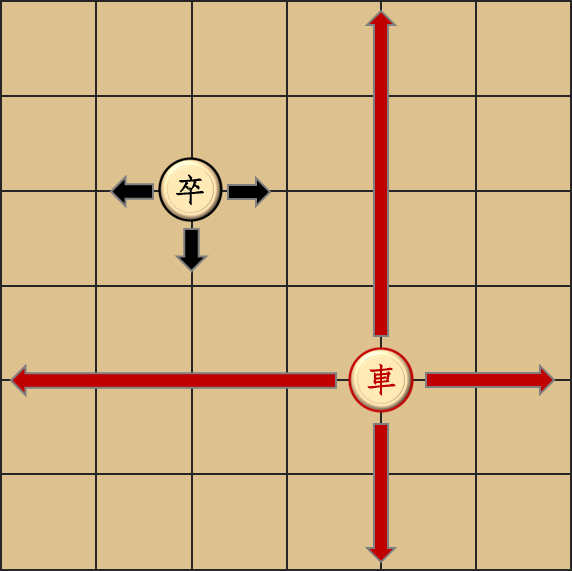1. 你操控车移动一次，也可以选择不动。
2. 如果车吃掉了卒（即车占据了卒所在位置），游戏结束。
3. 卒移动一步。当且仅当卒没有可移动方向时，卒才可以选择不动（即左、右、下三个方向均为车、毒气、棋盘边界中的一种）。例如，如果进行到某一轮前，$(1, 2)$ 有毒雾，且该回合车从 $(2, 2)$ 移动到 $(2, 1)$，那么卒无可移动方向，故卒该轮不进行移动。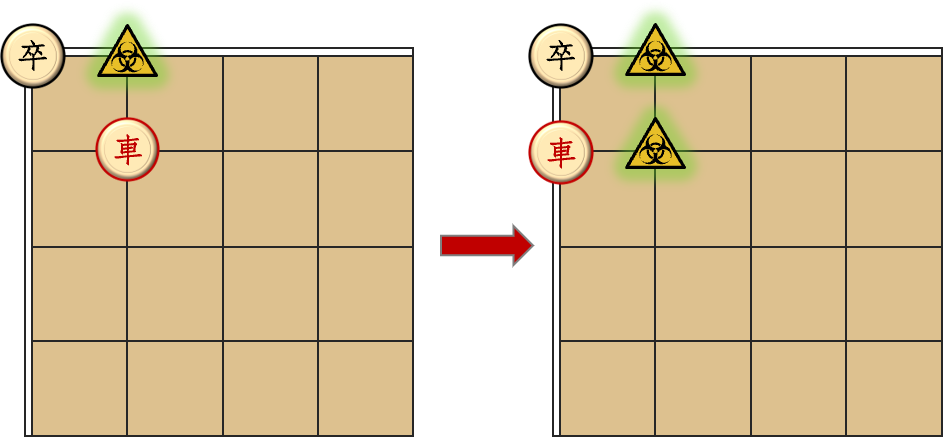### 输出格式

$T$ 行，每行一个整数表示游戏进行轮数。

### 样例一

#### input

4
1 1 2 2
1 2 2 4
100 50 3 3
50 2 49 4



#### output

2
3
2
3



#### explanation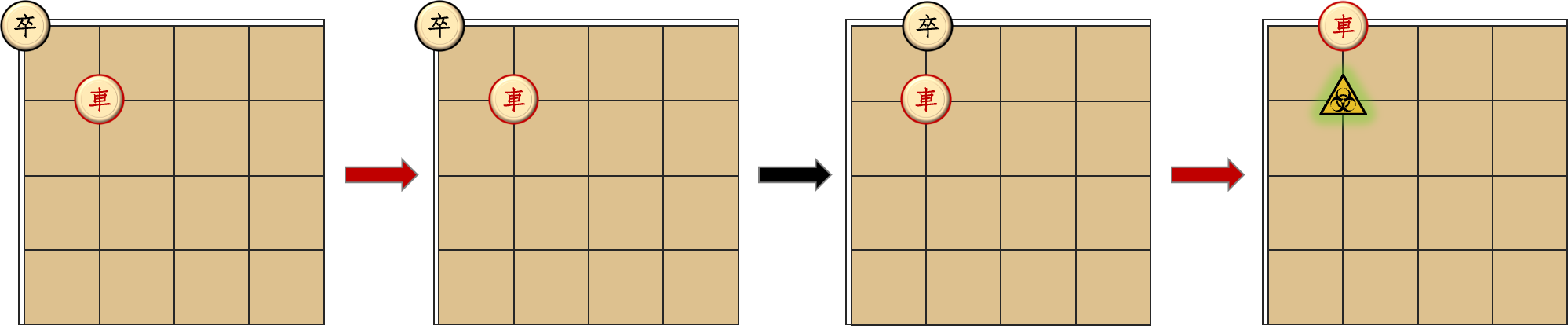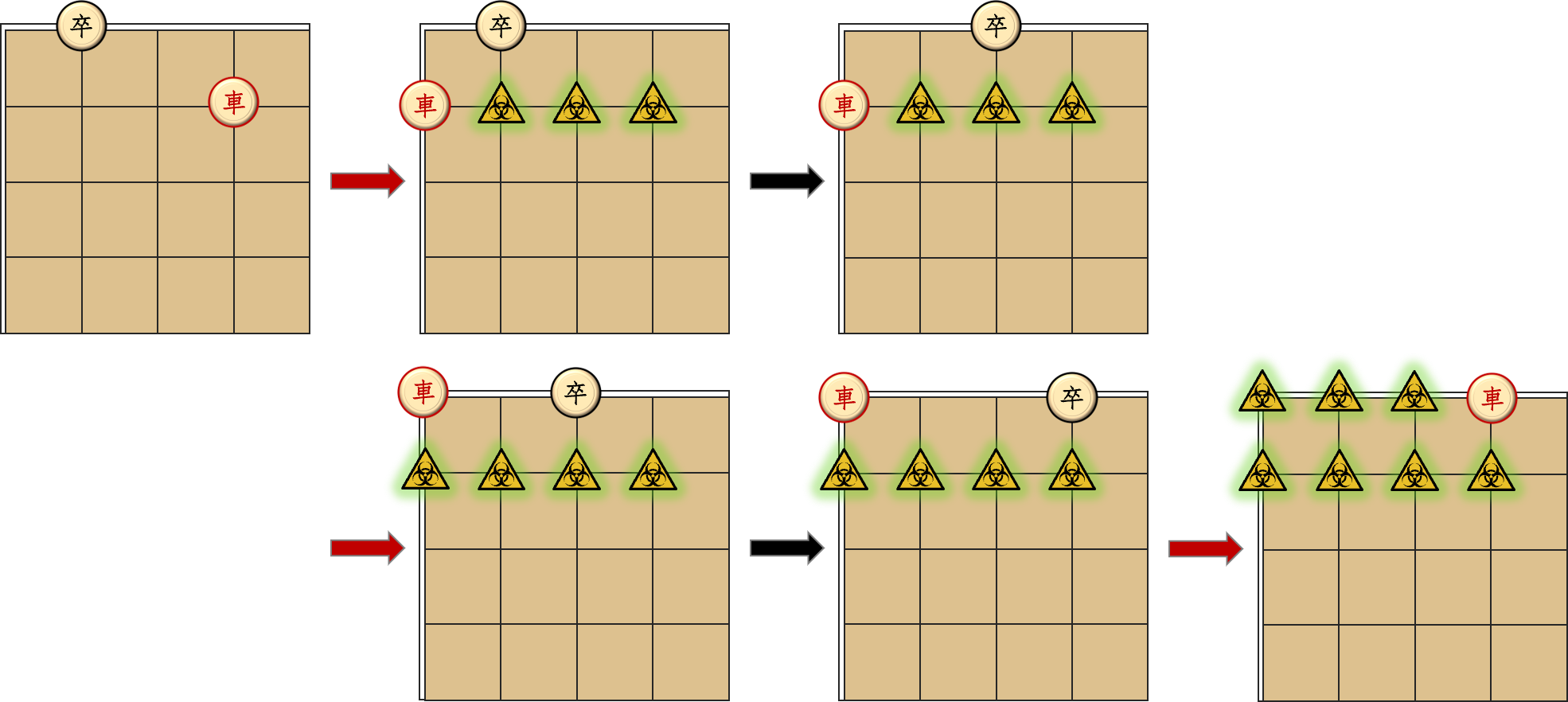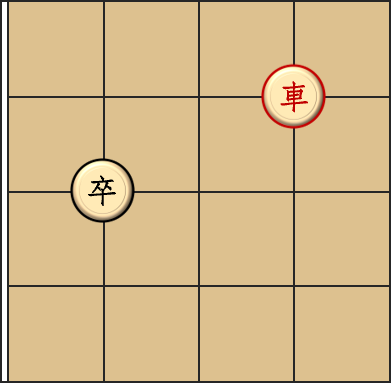### 样例二

#### input

13
72 75 73 56
59 55 60 56
59 70 59 65
82 66 82 62
97 2 98 6
97 2 98 15
63 79 64 82
57 71 58 75
96 64 97 66
96 73 97 76
96 99 95 97
96 99 88 98
98 100 100 99



#### output

3
3
1
1
3
3
3
3
3
3
3
3
2



### 数据范围与提示

$1$ $x_1=x_2$ $20$
$2$ $x_1=y_1=100$ $20$
$3$ $x_1=y_1=1$ $20$
$4$ $10\leq x_1,y_1,x_2,y_2\leq 90$ $20$
$5$ $20$